##### Electronics For Dummies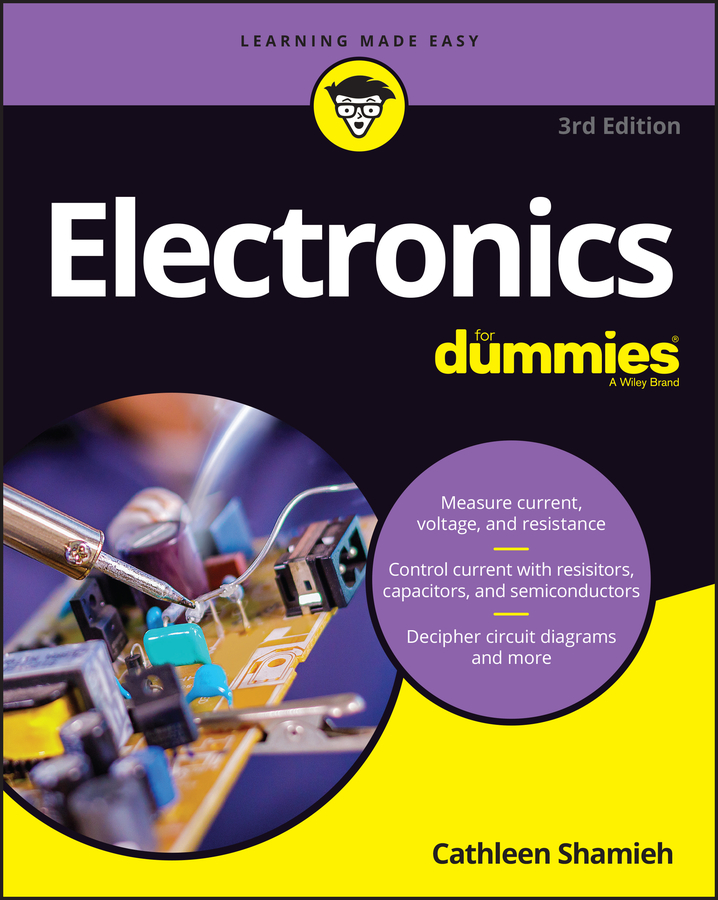A voltage comparator is an electronic circuit that compares two input voltages and lets you know which of the two is greater. It’s easy to create a voltage comparator from an op amp, because the polarity of the op-amp’s output circuit depends on the polarity of the difference between the two input voltages.

Suppose that you have a photocell that generates 0.5 V when it’s exposed to full sunlight, and you want to use this photocell as a sensor to determine when it’s daylight. You can use a voltage comparator to compare the voltage from the photocell with a 0.5 V reference voltage to determine whether or not the sun is shining.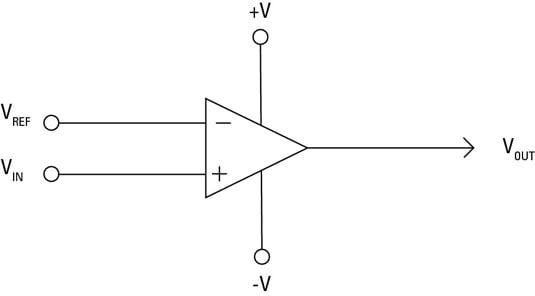In the voltage-comparator circuit, first a reference voltage is applied to the inverting input (V); then the voltage to be compared with the reference voltage is applied to the noninverting input. The output voltage depends on the value of the input voltage relative to the reference voltage, as follows:

Input Voltage Output Voltage
Less than reference voltage Negative
Equal to reference voltage Zero
Greater than reference voltage Positive

Note that the voltage level for both the positive and negative output voltages will be about 1 V less than the power supply. Thus, if the op-amp power supply is 9 V, the output voltage will be +8 V if the input voltage is greater than the reference voltage, 0 V if the input voltage is equal to the reference voltage, and –8 V if the input voltage is less than the reference voltage.

You can modify the circuit to eliminate the negative voltage if the input is less than the reference by sending the output through a diode. In this circuit, a positive voltage appears at the output if the input voltage is greater than the reference voltage; otherwise, no output voltage exists.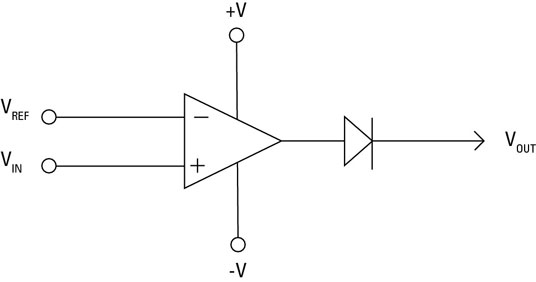To create a voltage comparator that creates a positive voltage output if the input voltage is less than a reference voltage, apply the reference voltage to the inverting (V) input, and the input voltage is applied to the noninverting (V+) input.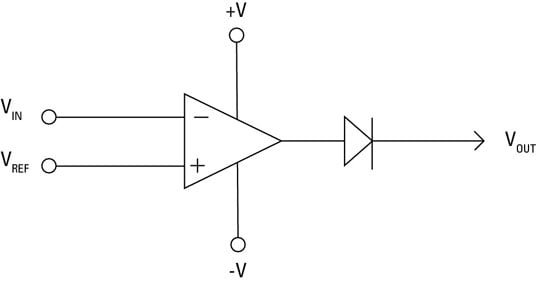The final voltage-comparator circuit you should know about is the window comparator, which lets you know whether the input voltage falls within a given range. A window comparator requires three inputs: a low reference voltage, a high reference voltage, and an input voltage.

The output of the window comparator will be a positive voltage only if the input voltage is greater than the low reference voltage and less than the high reference voltage. If the input voltage is less than the low reference voltage, the output will be zero. Similarly, if the input voltage is greater than the high reference voltage, the output will also be zero.

You need two op amps to create a window comparator. One op amp is configured to produce positive output voltage only if the input is greater than the low reference voltage (VREF(LOW)). The other op amp is configured to produce positive output voltage only if the input is less than the high reference voltage (VREF(HIGH)).

The input voltage is connected to both op amps; the output voltage is sent through diodes to allow only positive voltage and then combined. The resulting output will have positive voltage only if the input voltage falls between the low and high reference voltages.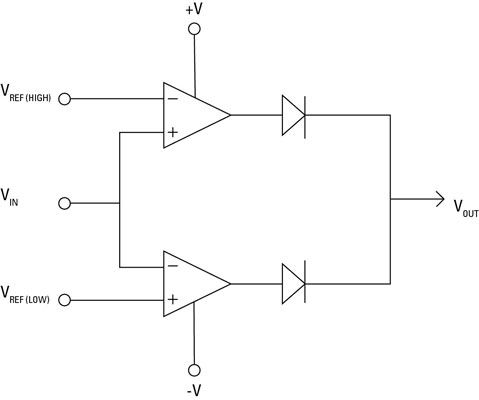Notice that the power supply connections aren’t shown separately for each op amp in the circuit. It’s common to omit the power supply connections when multiple op amps are used in a single circuit. If the power supply connections were shown for all of the op amps, the power supply connections would complicate the schematic unnecessarily.